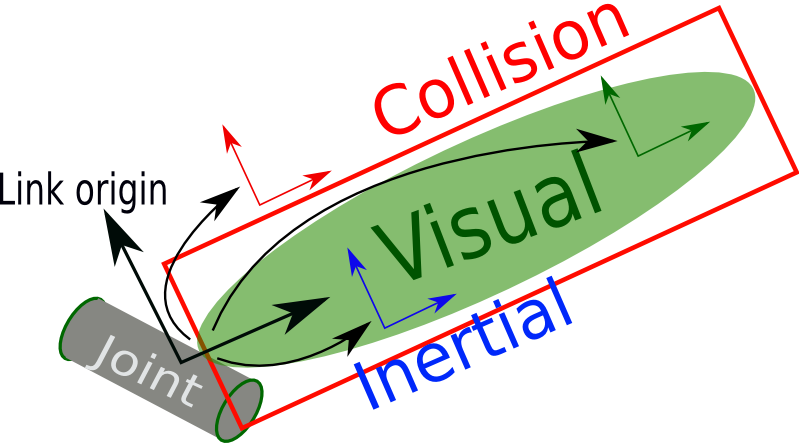[Documentation] [TitleIndex] [WordIndex]

The link element describes a rigid body with an inertia, visual features, and collision properties.

Here is an example of a link element:

```   1  <link name="my_link">
2    <inertial>
3      <origin xyz="0 0 0.5" rpy="0 0 0"/>
4      <mass value="1"/>
5      <inertia ixx="100"  ixy="0"  ixz="0" iyy="100" iyz="0" izz="100" />
6    </inertial>
7
8    <visual>
9      <origin xyz="0 0 0" rpy="0 0 0" />
10      <geometry>
11        <box size="1 1 1" />
12      </geometry>
13      <material name="Cyan">
14        <color rgba="0 1.0 1.0 1.0"/>
15      </material>
16    </visual>
17
18    <collision>
19      <origin xyz="0 0 0" rpy="0 0 0"/>
20      <geometry>
22      </geometry>
23    </collision>
```## Attributes

• name (required)

• The name of the link itself.

## Elements

• <inertial> (optional)

• The inertial properties of the link.

<origin> (optional: defaults to identity if not specified)

• This is the pose of the inertial reference frame, relative to the link reference frame. The origin of the inertial reference frame needs to be at the center of gravity. The axes of the inertial reference frame do not need to be aligned with the principal axes of the inertia.

• xyz (optional: defaults to zero vector)

• Represents theoffset.

rpy (optional: defaults to identity if not specified)

• Represents the fixed axis roll, pitch and yaw angles in radians.

<mass>

• The mass of the link is represented by the value attribute of this element

<inertia>

• The 3x3 rotational inertia matrix, represented in the inertia frame. Because the rotational inertia matrix is symmetric, only 6 above-diagonal elements of this matrix are specified here, using the attributes ixx, ixy, ixz, iyy, iyz, izz.

<visual> (optional)

• The visual properties of the link. This element specifies the shape of the object (box, cylinder, etc.) for visualization purposes. Note: multiple instances of <visual> tags can exist for the same link. The union of the geometry they define forms the visual representation of the link.

name (optional)

• Specifies a name for a part of a link's geometry. This is useful to be able to refer to specific bits of the geometry of a link.

<origin> (optional: defaults to identity if not specified)

• The reference frame of the visual element with respect to the reference frame of the link.

xyz (optional: defaults to zero vector)

• Represents theoffset.

rpy (optional: defaults to identity if not specified)

• Represents the fixed axis roll, pitch and yaw angles in radians.

<geometry> (required)

• The shape of the visual object. This can be one of the following:

• <box>

• size attribute contains the three side lengths of the box. The origin of the box is in its center.

<cylinder>

• Specify the radius and length. The origin of the cylinder is in its center.<sphere>

• Specify the radius. The origin of the sphere is in its center.

<mesh>

• A trimesh element specified by a filename, and an optional scale that scales the mesh's axis-aligned-bounding-box. The recommended format for best texture and color support is Collada .dae files, though .stl files are also supported. The mesh file is not transferred between machines referencing the same model. It must be a local file.

<material> (optional)

• The material of the visual element. It is allowed to specify a material element outside of the 'link' object, in the top level 'robot' element. From within a link element you can then reference the material by name.
• name name of the material

• <color> (optional)

• rgba The color of a material specified by set of four numbers representing red/green/blue/alpha, each in the range of [0,1].

<texture> (optional)

• The texture of a material is specified by a filename

<collision> (optional)

• The collision properties of a link. Note that this can be different from the visual properties of a link, for example, simpler collision models are often used to reduce computation time. Note: multiple instances of <collision> tags can exist for the same link. The union of the geometry they define forms the collision representation of the link.

name (optional)

• Specifies a name for a part of a link's geometry. This is useful to be able to refer to specific bits of the geometry of a link.

<origin> (optional: defaults to identity if not specified)

• The reference frame of the collision element, relative to the reference frame of the link.

xyz (optional: defaults to zero vector)

• Represents theoffset.

rpy (optional: defaults to identity if not specified)

• Represents the fixed axis roll, pitch and yaw angles in radians.

<geometry>

• See the geometry description in the above visual element.

• For collision checking using the ROS motion planning packages, as few faces per link as possible are recommended for the collision meshes that you put into the URDF (ideally less than 1000). If possible, approximating the meshes with other primitives is encouraged.

## Multiple Collision Bodies

It was decided that URDFs should not support multiple groups of collision bodies, even though there are sometimes applications for this. The URDF is intended to only represent the actual robot's properties, and not collisions used for external things like controller collision checking. In a URDF, the <visual> elements should be as accurate as possible to the real robot, and the <collision> elements should still be a close approximation, albeit with far fewer triangles in the meshes.

If you do need courser-grain, over sized collision geometries for things like collision checking and controllers, you can move these meshes/geometries to custom XML elements. For example, if your controllers need some special rough collision checking geometry, you could add the tag <collision_checking> after the <collision> element:

```  <link name="torso">
<visual>
<origin rpy="0 0 0" xyz="0 0 0"/>
<geometry>
</geometry>
</visual>
<collision>
<origin rpy="0 0 0" xyz="-0.065 0 0.0"/>
<geometry>
</geometry>
</collision>
<collision_checking>
<origin rpy="0 0 0" xyz="-0.065 0 0.0"/>
<geometry>